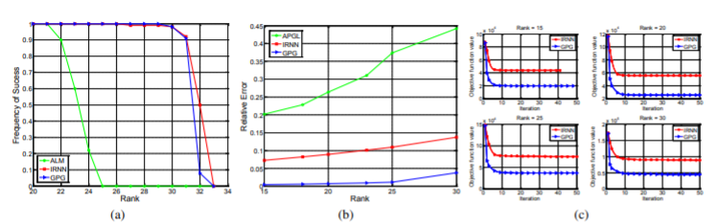# Generalized Singular Value Thresholding### Abstract

This work studies the Generalized Singular Value Thresholding (GSVT) operator associated with a nonconvex function g defined on the singular values of X. We prove that GSVT can be obtained by performing the proximal operator of g (denoted as Proxg(·)) on the singular values since Proxg(·) is monotone when g is lower bounded. If the nonconvex g satisfies some conditions (many popular nonconvex surrogate functions, e.g., ‘p-norm, 0 < p < 1, of ‘0-norm are special cases), a general solver to find Proxg(b) is proposed for any b \geq 0. GSVT greatly generalizes the known Singular Value Thresholding (SVT) which is a basic subroutine in many convex low rank minimization methods. We are able to solve the nonconvex low rank minimization problem by using GSVT in place of SVT.

Type
Publication
AAAI Conference on Artificial Intelligence